Share

# Lakhmir Singh solutions for Class 10 Physics chapter 2 - Magnetic Effects of Electric Current [Latest edition]

Course
Textbook page

#### Chapters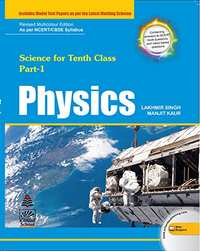## Chapter 2: Magnetic Effects of Electric Current

#### Lakhmir Singh solutions for Class 10 Physics Chapter 2 Magnetic Effects of Electric Current [Pages 0 - 82]

Q 1

Write any three properties of magnetic lines of force.

Q 2

What are the two ways in which you can trace the magnetic field pattern of a bar magnet?

Q 3 | Page 73

You are given the magnetic field pattern of a magnet. How will you find out from it where the magnetic field is the strongest?

Q 4 | Page 73

State whether the following statement is true of false:
The axis of earth's imaginary magnet and the geographical axis coincide with each other.

Q 4 | Page 82

State qualitatively the effect of inserting an iron core into a current-carrying solenoid.

Q 5 | Page 73

Why does a compass needle get deflected when brought near a bar magnet?

Q 6 | Page 73

Where do the manufactures use a magnetic strip in the refrigerator? Why is this magnetic strip used?

Q 7.1

Fill in the following blank with suitable words:

Magnetic field lines leave the .......... pole of a bar magnet and enter at its .......... pole.

Q 7.2

Fill in the following blank with suitable words:

The earth's magnetic field is rather like that of a .......... magnet with its .......... pole in the northern hemisphere.

Q 8 | Page 73

Draw a diagram to show the magnetic field lines around a bar magnet.

Q 9 | Page 73

What is a magnetic field? How can the direction of magnetic field lines at a place be determined?

Q 10 | Page 73

Explain why, two magnetic field lines of not intersect each other.

Q 11 | Page 73

When an electric current is passed through any wire, a magnetic field is produced around it. Then why an electric iron connecting cable does not attract nearby iron objects when electric  current switched on through it?

Q 12.1 | Page 73

Define magnetic field lines. Describe an activity to draw a magnetic field line outside a bar magnet from one pole to another pole.

Q 12.2 | Page 73

Explain why, a freely suspended magnet always points in the north-south direction.

Q 13 | Page 73

A strong bar magnet is placed vertically above a horizontal wooden board. The magnetic lines of force will be:

(a) only in horizontal plane around the magnet
(b) only in vertical plane around the magnet
(c) in horizontal as well as in vertical planes around the magnet
(d) in all the planes around the magnet

Q 14 | Page 73

The magnetic field lines produced by a bar magnet:

(a) originate from the south pole and end at its north pole
(b) originate from the north pole and end at its east pole
(c) originate from the north pole and end at its south pole
(d) originate from the south pole and end at its west pole

Q 15 | Page 73

Which of the following is not attracted by a magnet:

(a) steel
(b) cobalt
(c) brass
(d) nickel

Q 16 | Page 73

The magnetic field lines:

(a) intersect at right angles to one another
(b) interest at an angle 45° to each another
(c) do not cross one another
(d) cross at an angle of 60° to one anotherv

Q 19 | Page 74

The shape of the earth's magnetic field resembles that of an imaginary:

(a) U-shaped magnet
(b) Straight conductor carrying current
(c) Current-carrying circular coil
(d) Bar magnet

Q 20 | Page 74

A magnet attracts:

(a) plastics
(b) any metal
(c) aluminium
(d) iron and steel

Q 21 | Page 74

A plotting compass is placed near the south pole of a bar magnet. The pointer of plotting compass will:

(a) point away form the south pole
(b) point parallel to the south pole
(c) point towards the south pole
(d) point at right angles to the south pole

Q 22 | Page 73

The metallic pointer of a plotting compass gets deflected only when it is placed near a bar magnet because the pointer has:

(a) electromagnetism
(b) permanent magnetism
(c) induced magnetism
(d) ferromagnetism

Q 23 | Page 74

Which of the following statements is incorrect regarding magnetic field lines?
(a) The direction of magnetic field at a point is taken to be the direction in which the north pole a magnetic compass needle points.
(b) Magnetic field lines are closed curves.
(c) If magnetic field lines are parallel and equidistant, they represent zero field strength.
(d) Relative strength of magnetic field is shown by the degree of closeness of the field lines.

Q 24 | Page 74

Copy the figure below which shows a plotting compass and a magnet. Label the N pole of the magnet and draw field line on which the compass lines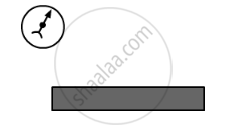Q 25 | Page 74

The diagram shows a bar magnet surrounded by four plotting compasses. Copy the diagram and mark in it the direction of he compass needle for each of the cases B, C and D.

Which is the north pole, X or Y?

Q 26 | Page 74

The three diagrams in the following figure show the lines of force (field lines) between the poles of two magnets. Identify the poles A, B, C, D, E and F.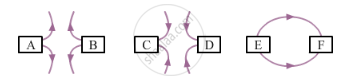Q 27 | Page 74

The figure given below shows the magnetic field between two magnets: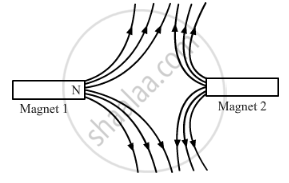Copy the diagram and label theother poles of the magnets.

Which is the weaker magnet?

#### Lakhmir Singh solutions for Class 10 Physics Chapter 2 Magnetic Effects of Electric Current [Pages 18 - 92]

Q 1 | Page 81

Which effect of current can be utilised in detecting a current carrying wire concealed in a wall?

Q 2 | Page 81

What concealed do you get from the observation that a current-carrying wire deflects a compass needle placed near it?

Q 3 | Page 82

Name the scientist who discovered the magnetic effect of current.

Q 5 | Page 82

Name the rule for finding the direction of magnetic field produced by a straight current-carrying conductor.

Q 6 | Page 82

State the form of magnetic field lines around a straight current-carrying conductor.

Q 7 | Page 82

What is the other name of Maxwell's right-hand thumb rule?

Q 8 | Page 82

State whether the following statement is true or false:
The magnetic field inside a long circular coil carrying current well be parallel straight lines.

Q 9 | Page 82

What is the shape of a current-carrying conductor whose magnetic field pattern resembles that of a bar magnet?

Q 10 | Page 82

State three ways in which the strength of an electromagnet can be increased.

Q 11.1 | Page 82

Fill in the following blank with suitable words:

The lines of ........... round a straight current-carrying conductor are in the shape of ...........

Q 11.2 | Page 82

Fill in the following blank with suitable words:

For a current-carrying solenoid, the magnetic field is like that of a ...........

Q 11.3 | Page 82

Fill in the following blank with suitable words:

The magnetic effect of a coil can be increased by increasing the number of ........... increasing the ........... or inserting an ........... core.

Q 11.4 | Page 82

Fill in the following blank with suitable words:

If a coil is viewed from one end and the current flows in an anticlockwise direction, then this end is a ........... pole.

Q 11.5 | Page 82

Fill in the following blank with suitable words:

If a coil is viewed from one end, and the current flows in a clockwise direction, then this end is a ........... pole.

Q 12 | Page 82

Describe how you will locate a current-carrying wire concealed in a wall.

Q 13 | Page 82

Describe some experiment to show that the magnetic field is associated with an electric current.

Q 14.1 | Page 82

Draw a sketch to show the magnetic lines of force due to a current-carrying straight conductor.

Q 14.2 | Page 82

Name and state the rule to determine the direction of magnetic field around a straight current-carrying conductor.

Q 15 | Page 82

State and explain Maxwell's right-hand thumb rule.

Q 16 | Page 82

What is Maxwell's corkscrew rule? For what purpose is if used?

Q 17.1 | Page 82

Draw the magnetic lines of force due to a circular wire carrying current.

Q 17.2 | Page 82

What are the various ways in which the strength of magnetic field produced by a current-carrying circular coil can be increased?

Q 18 | Page 82

State and explain the Clock face rule for determining the polarities of a circular wire carrying current.

Q 19 | Page 82

Name any two factors on which the strength of magnetic field produced by a current-carrying solenoid depends. How does it depend on these factors?

Q 20.1 | Page 82

Draw a circuit diagram to show how a soft iron piece can be transformed into an electromagnet.

Q 20.2 | Page 82

Describe how an electromagnet could be used to separate copper from iron in a scrap yard.

Q 21.1 | Page 82

How does an electromagnet differ forma permanent magnet?

Q 21.2 | Page 82

Name two devices in which electromagnets are used and two devices where permanent magnets are used.

Q 22.1 | Page 82

What is a solenoid? Draw a sketch to show the magnetic field pattern produced by a current-carrying solenoid.

Q 22.2 | Page 82

Name the type of magnet with which the magnetic field pattern of a current-carrying solenoid resembles

Q 22.3 | Page 82

What is the shape of field lines inside a current-carrying solenoid? What does the pattern of field lines inside a current-carrying solenoid indicate?

Q 22.4 | Page 82

List three ways in which the magnetic field strength of a current-carrying solenoid can be increased?

Q 22.5 | Page 82

What type of core should be put inside a current-carrying solenoid to make an electromagnet?

Q 23.1 | Page 82

What is an electromagnet? Describe the construction and working of an electromagnet with the help of a labelled diagram.

Q 23.2 | Page 82

Explain why, an electromagnet is called a temporary magnet.

Q 23.3 | Page 82

Explain why, the core of an electromagnet should be of soft iron and not of steel.

Q 23.4 | Page 82

State the factors on which the strength of an electromagnet depends. How does it depend on these factors?

Q 23.5 | Page 18

Write some of the important uses of electromagnets.

Q 24 | Page 83

The strength of the magnetic field between the poles of an electromagnet would be unchanged if:

Q 24 | Page 92

An electron beam enters a magnetic field at right angles to it as shown in the Figure.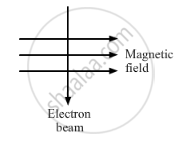The direction of force acting on the electron beam will be:

to the left
to the right
into the page
out of the page

Q 25 | Page 83

The diagram given below represents magnetic field caused by a current-carrying conductor which is: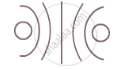(a) a long straight wire
(b) a circular coil
(c) a solenoid
(d) a short straight wire

Q 26 | Page 83

The magnetic field inside a long straight solenoid carrying current:

(a) is zero
(b) decreases as we move towards its end.
(c) increases as we move towards its end.
(d) is the same at all points.

Q 27 | Page 83

Which of the following correctly describes the magnetic field near a long straight wire?

• The field consists of straight lines perpendicular to the wire

• The field consists of straight lines parallel to the wire

• The field consists of radial lines originating from the wire

• The field consists of concentric circles centred on the wire

Q 28 | Page 83

The north-south polarities of an electromagnet can be found easily by using:

(a) Fleming's right-hand rule
(b) Fleming's left-hand rule
(c) Clock face rule
(d) Left-hand thumb rule

Q 29 | Page 83

The direction of current in the coil at one end of an electromagnet is clockwise. This end of the electromagnet will be:

(a) north pole
(b) east pole
(c) south pole
(d) west pole

Q 30 | Page 83

If the direction of electric current in a solenoid when viewed from a particular end is anticlockwise, then this end of solenoid will be:

(a) west pole
(b) south pole
(c) north pole
(d) east pole

Q 31 | Page 83

The most suitable material for making the core of an electromagnet is:

(a) soft iron
(b) brass
(c) aluminium
(d) steel

Q 32 | Page 83

The magnetic effect of current was discovered by:

(a) Maxwell
(b) Fleming
(c) Oersted

Q 33 | Page 83

A soft iron bar is inserted inside a current-carrying solenoid. The magnetic field inside the solenoid:

(a) will decrease
(b) will increase
(c) will become zero
(d) will remain the same

Q 34 | Page 83

The magnetic field lines in the middle of the current-carrying solenoid are?

(a) circles
(b) spirals
(c) parallel to the axis of the tube
(d) perpendicular to the axis of the tube

Q 35 | Page 83

The front face of a circular wire carrying current behaves like a north pole. The direction of current in this face of circular wire is:

(a) clockwise
(b) downwards
(c) anticlockwise
(d) upwards

Q 36 | Page 84

The back face of a circular loop of wire is found to be south magnetic pole. The direction of current in this face of the circular loop of wire will be:

(a) towards south
(b) clockwise
(c) anticlockwise
(d) towards north

Q 37 | Page 84

In the straight wire A, current is flowing in the vertically downwards direction whereas in wire B the current is flowing in the vertically upward direction. What is the direction of magnetic field:

(a) in wire A?
(b) in wire B?
Name the rule which you have used to get the answer.

Q 38 | Page 84

The figure shows a solenoid wound on a core of soft iron. Will the end A be a N pole or S pole when the current flows in the direction shown?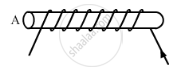Q 39 | Page 84

A current-carrying straight wire is held in exactly vertical position. If the current passes through this wire in the vertically upward direction, what is the direction of magnetic field produced by it? Name the rule used to find the direction of magnetic field.

Q 40 | Page 84

For the coil in the diagram below, when the switch is pressed:

(a) what is the polarity of end A?
(b) which way will the compass point then?Q 41 | Page 84

A current flows downwards in a wire that passes vertically through a table top. Will the magnetic field lines around it go clockwise or anticlockwise when viewed from above the table?

Q 42 | Page 84

The direction of current flowing in the coil of an electromagnet at its two ends X and Y are as shown below: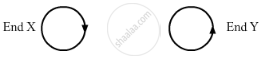What is the polarity of end X?

What is the polarity of end Y?

Name and state the rule which you have used to determine the polarities.

Q 43 | Page 84

The magnetic field associated with a current-carrying straight conductor is in anticlockwise direction. If the conductor was held along the east-west direction, what will be the direction of current through it? Name and state the rule applied to determine the direction of current?

Q 44 | Page 84

A current-carrying conductor is held in exactly vertical direction. In order to produce a clockwise magnetic field around the conductor, the current should passed in the conductor:

(a) from top towards bottom
(b) from left towards right
(c) from bottom towards top
(d) from right towards left

Q 45 | Page 84

A thick wire is hanging form a wooden table. An anticlockwise magnetic field is to be produced around the wire by passing current through this wire by using a battery. Which terminal of the battery should be connected to the:

(a) top end of wire?
(b) bottom end of wire?

#### Lakhmir Singh solutions for Class 10 Physics Chapter 2 Magnetic Effects of Electric Current [Pages 91 - 93]

Q 1 | Page 91

What happens when a current-carrying conductor is placed in a magnetic field?

Q 2 | Page 91

When is the force experienced by a current-carrying conductor placed in a magnetic field largest?

Q 3 | Page 91

In a statement of Fleming's left-hand rule, what do the following represent?

(a) direction of centre finger.
(b) direction of forefinger.
(c) direction of thumb.

Q 4 | Page 91

Name one device which works on the magnetic effect of current.

Q 5 | Page 91

Electric motor works on the magnetic effect of electric current.

Q 6 | Page 91

A motor converts one form of energy into another. Name the two forms.

Q 7 | Page 91

State whether the following statement is true or false:
An electric motor converts mechanical energy into electrical energy.

Q 8 | Page 91

For Fleming's left-hand rule, write down the three things that are 90° to each other, and next to each one write down the finger or thumb that represents it.

Q 9 | Page 91

Name the device which is used to reverse the direction of current in the coil of a motor?

Q 10 | Page 91

What is the other name of the split ring used in an electric motor?

Q 11 | Page 91

What is the function of a commutator in an electric motor made ?

Q 12 | Page 91

Of what substance are the brushes of an electric motor made?

Q 13 | Page 91

Of what substance is the core of the coil of an electric motor made?

Q 14 | Page 91

In an electric motor, which of the following remains fixed and which rotates with the coil?
Commutator ; Brush

Q 15 | Page 91

What is the role of the spilt ring in an electric motor?

Q 16.1 | Page 91

Fill in the following blank with suitable words:

Fleming's Rule for the motor effect uses the .......... hand.

Q 16.2 | Page 91

Fill in the following blank with suitable words:

A motor contains a kind of switch called a .......... which reverses the current every half .......... .

Q 17.1 | Page 91

A current-carrying conductor is placed perpendicularly in a magnetic field. Name the rule which can be used to find the direction of force acting on the conductor.

Q 17.2 | Page 91

State two ways to increase the force on a current-carrying conductor in a magnetic field.

Q 17.3 | Page 91

Name one device whose working depends on the force exerted on a current-carrying coil placed in a magnetic field.

Q 18 | Page 92

State Fleming's left-hand rule. Explain it with the help of labelled diagrams.

Q 19.1 | Page 92

What is the principle of an electric motor?

Q 19.2 | Page 92

Name some of the devices in which electric motor are used.

Q 20.1 | Page 92

In a d.c. motor, why must the current to the coil be reversed twice during each rotation?

Q 20.2 | Page 92

What device reverses the current?

Q 21.1 | Page 92

State what would happen to the direction of rotation of a motor if:
the current were reversed

Q 21.1 | Page 92

State what would happen to the direction of rotation of a motor if:

both current and magnetic field were reversed simultaneously.

Q 21.2 | Page 92

State what would happen to the direction of rotation of a motor if:

In what ways can a motor be made more powerful?

Q 22.1 | Page 92

What is an electric motor? With the help of a labelled diagram, describe the working of a simple electric motor

Q 22.2 | Page 92

What are the special features of commercial electric motors?

Q 23 | Page 92

In an electric motor, the direction of current in the coil changes once in each:
two rotations
one rotation
half rotation
one-four rotation

Q 25 | Page 92

The force experienced by a current-carrying conductor placed in a magnetic field is the largest when the angle between the conductor and the magnetic field is:

45°
60°
90°
180°

Q 26 | Page 92

The force exerted on a current-carrying wire placed in a magnetic field is zero when the angle between the wire and the direction of magnetic field is:

45°
60°
90°
180°

Q 27 | Page 92

A current flows in a wire running between the S and N poles of a magnet lying horizontally as shown in Figure below:

The force on the wire due to the magnet is directed: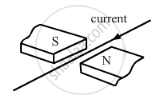fron N to S
from S to N
vertically downwards
vertically upwards

Q 28 | Page 92

An electric motor is a device which transforms:

mechanical energy to electrical
heat energy to electrical energy
electrical energy to heat energy only
electrical energy to mechanical energy

Q 29 | Page 92

A magnetic field exerts no force on:

an electrical charge moving perpendicular to its direction
an unmagnetised iron bar
a stationary electric charge
a magnet

Q 30 | Page 93

A horizontal wire carries a current as shown in Figure below between magnetic poles N and S: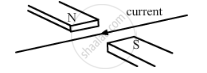Is the direction of the force on the wire due to the magnet:

(a) in the direction the current
(b) vertically downwards
(c) opposite to the current direction
(d) vertically upwards

Q 31 | Page 93

In the simple electric motor of figure given below, the coil rotates anticlockwise as seen by the eye from the position X when current flows in the coil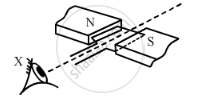Is the current flowing clockwise or anticlockwise around the coil when viewed from above?

Q 32 | Page 93

Which way does the wire in the diagram below  tend to move?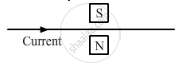Q 33 | Page 93

If the current in a wire is flowing in the vertically downward direction and a magnetic field is applied from west to east, what is the direction of force in the wire?

Q 34 | Page 93

Which way does the wire in the diagram below tend to move?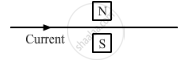Q 35 | Page 93

What is the force on a current-carrying wire that is parallel to a magnetic field? Give reason for your answer.

Q 36 | Page 93

A charged particle enters at right angles into a uniform magnetic field as shown: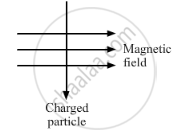What should be the nature of charge on the particle if it begins to move in a direction pointing vertically our of the page due to its interaction with the magnetic field?

#### Lakhmir Singh solutions for Class 10 Physics Chapter 2 Magnetic Effects of Electric Current [Pages 102 - 108]

Q 1 | Page 102

Name the device which converts mechanical energy into electric energy.

Q 2 | Page 102

Out of an A.C. generator and a D.C. generator:

which one uses a commutator (split rings)?
which one uses slip rings?

Q 3 | Page 102

Name the phenomenon which is made use of in an electric generator.

Q 4 | Page 102

Name the rule which gives the direction of induced current.

Q 5 | Page 102

What condition is necessary for the production of current by electromagnetic induction?

Q 6 | Page 103

What type of generator is used at Power Stations?

Q 7 | Page 103

What change should be made in an a.c. generator so that it may become a d.c. generator?

Q 8.1 | Page 103

State whether the following statement are true or false:

A generator works on the principle of electromagnetic induction.

Q 8.2 | Page 103

State whether the following statement are true or false:

A motor works on the principle electric generator?

Q 9 | Page 103

What is the function of brushes in an electric generator?

Q 10 | Page 103

When a wire is moved up and down in a magnetic field, a current is induced in the wire. What is this phenomenon known as?

Q 11 | Page 103

When current is 'switched on' and 'switched off' in a coil, a current is induced in another coil kept near it. What is this phenomenon known as?

Q 12 | Page 103

What is the major difference between the simple alternator and most practical alternators?

Q 13 | Page 103

Why are Thermal Power Stations usually located near a river?

Q 14 | Page 103

List three sources of magnetic fields.

Q 15 | Page 103

Complete the following sentence:

A generator with commutator produces ............... current.

Q 16 | Page 103

Two circular coils A and B are placed closed to each other. If the current in the coil A is changed, will some current be induced in the coil B? Give reason.

Q 17.1 | Page 103

Explain the principle of an electric generator.

Q 17.2 | Page 103

State two ways in the which the current alternating current and direct current?

Q 18.1 | Page 103

What is the difference between alternating current and direct current?

Q 18.2 | Page 103

What type of current is given by (i) a dry cell, and (ii) a power House generator?

Q 19 | Page 103

State and explain Fleming's right hand rule.

Q 20.1 | Page 103

Name and state the rule to find the direction of:

current induced in a coil due to its rotation in a magnetic field.

Q 20.2 | Page 103

force experienced by a current-carrying straight conductor placed in a magnetic field which is perpendicular to it.

Q 21.1 | Page 103

In what respect does the construction of an A.C. generator differ from that of a D.C. generator?

Q 21.2 | Page 103

What normally drivers the alternators in a Thermal Power Station? What fuels can be used to heat eater in the boiler?

Q 22 | Page 103

Draw the labelled diagram of an A.C. generator. With the help of this diagram, explain the construction and working of an A.C. generator.

Q 23.1 | Page 103

What do you understand by the term "electromagnetic induction"? Explain with the help of a diagram.

Q 23.2 | Page 103

Name one device which works on the phenomenon of electromagnetic induction.

Q 23.3 | Page 103

Describe different ways to induce current in a coil of wire.

Q 24.1 | Page 103

What do you understand by the terms 'direct current' and 'alternating current'?

Q 24.2 | Page 103

Name some sources of direct current and some of alternating current.

Q 24.3 | Page 103

State an important advantage of alternating current over direct current.

Q 24.4 | Page 103

What is the frequency of A.C. supply in India?

Q 25 | Page 103

A rectangular coil of copper wires is rotated in a magnetic field. The direction of the induced current changes once in each

• two revolutions

• one revolution

• half revolution

• one-fourth revolution

Q 26 | Page 103

The phenomenon of electromagnetic induction is

• the process of charging a body

• the process of generating magnetic field due to a current passing through a coil

• producing induced current in a coil due to relative motion between a magnet and the coil

• the process of rotating a coil of an electric motor

Q 27 | Page 104

The device used for producing electric current is called a:

(a) generator
(b) galvanometer
(c) ammeter
(d) motor

Q 28 | Page 104

The essential difference between an AC generator and a DC generator is that

• AC generator has an electromagnet while a DC generator has permanent magnet.

• DC generator will generate a higher voltage.

• AC generator will generate a higher voltage.

• AC generator has slip rings while the DC generator has a commutator.

Q 29 | Page 104

When the switch S is closed in the figure given below, the pointer of the galvanometer moves to the right.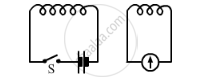If S is kept closed, will the pointer:

(b) stay over on the right?
(c) move to the left and stay there
(d) move to and fro until S is opened

Q 30 | Page 104

Each one of the following changes will increase emf (or voltage) in a simple generator except:

(a) increasing the number of turns in the armature coil
(b) winding the coil on a soft iron armature
(c) increasing the size of the gap in which the armature turns
(d) increasing the speed of rotation

Q 31 | Page 104

The north pole of a long bar magnet was pushed slowly into a short solenoid connected to a galvanometer. The magnet was held stationary for a few seconds with the north pole in the middle of the solenoid and then withdrawn rapidly. The maximum deflection of the galvanometer was observed when the magnet was:

(a) moving towards the solenoid
(b) moving into solenoid
(c) at rest inside the solenoid
(d) moving out of the solenoid

Q 32 | Page 104

An electric generator converts:

(a) electrical energy into mechanical energy.
(b) mechanical energy into heat energy.
(c) electrical energy into chemical energy.
(d) mechanical energy into electrical energy.

Q 33 | Page 104

A d.c. generator is based on the principle of:

(a) electrochemical induction
(b) electromagnetic induction
(c) magnetic effect of current
(d) heating effect of current

Q 34 | Page 104

An induced current is produced when a magnet is moved into a coil. The magnitude of induced current does not depend on:

(a) the speed with which the magnet is moved
(b) the number of turns of the coil
(c) the resistivity of the wire of the coil
(d) the strength of the magnet

Q 36 | Page 104

The frequency of direct current (d.c.) is:

(a) 0 Hz
(b) 50 Hz
(c) 60 Hz
(d) 100 H

Q 36 | Page 104

The frequency of alternating current (a.c.) supply in India is:

(a) 0 Hz
(b) 50 Hz
(c) 60 Hz
(d) 100 Hz

Q 37.1 | Page 105

A coil is connected to a galvanometer. When the N-pole of magnet is pushed into the coil, the galvanometer deflected to the right. What deflection, if any, is observed when:

the N-pole is removed?

Q 37.2 | Page 105

A coil is connected to a galvanometer. When the N-pole of magnet is pushed into the coil, the galvanometer deflected to the right. What deflection, if any, is observed when:

the S-pole is inserted?

Q 37.3 | Page 105

A coil is connected to a galvanometer. When the N-pole of magnet is pushed into the coil, the galvanometer deflected to the right. What deflection, if any, is observed when:

the magnet is a rest in the coil?

Q 38 | Page 105

When the magnet shown in the diagram below is moving towards the coil, the galvanometer gives a reading to the right.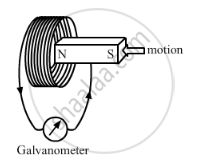() What is the name of the effect being produced by the moving magnet?
(2) State what happens to the reading shown on the galvanometer when the magnet is moving away from the coil.
(3) The original experiment is repeated. This time the magnet is moved towards the coil at a great speed. State two changes you would notice in the reading on the galvanometer.

Q 39 | Page 105

If you hold a coil of wire next to a magnet, no current will flow in the coil. What else is needed to induce a current?

Q 40 | Page 108

The wire in Figure below is being moved downwards through the magnetic field so as to produce induced current.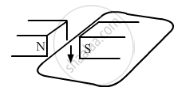(a) moving the wire at a higher speed?
(b) moving the wire upwards rather than downwards?
(c) using a stronger magnet?
(d) holding the wire still in the magnetic field?
(e) moving the wire parallel to the magnetic field lines?

Q 41.1 | Page 105

Two coils A and B of insulated wire are kept close to each other. Coil A is connected to a galvanometer while coil B is connected to a battery through a key. What would happen if:

a current is passed through coil B by plugging the key?

Q 41.2 | Page 105

Two coils A and B of insulated wire are kept close to each other. Coil A is connected to a galvanometer while coil B is connected to a battery through a key. What would happen if:

the current is stopped by removing the plug from the key?

Q 42 | Page 105

A portable radio has a built-in transformer so that it can work from the mains instead of batteries. Is this a set-up or step down transformer?

#### Lakhmir Singh solutions for Class 10 Physics Chapter 2 Magnetic Effects of Electric Current [Pages 113 - 114]

Q 1 | Page 113

What name is given to the device which automatically cuts off the electricity supply during short-circuiting in household wiring?

Q 2.1 | Page 113

What is the usual capacity of an electric fuse used (i) in the lighting circuit

Q 2.2 | Page 113

What is the usual capacity of an electric fuse used  in the power circuit, of a small house?

Q 3 | Page 113

Give the symbol of an electric fuse used in circuit diagrams.

Q 4.1 | Page 113

State whether the following statement are true of false:

A wire with a green insulation is usually the live wire.

Q 4.2 | Page 113

State whether the following statement are true of false:

A miniature circuit breaker (MCB) works on the heating effect of current.

Q 5 | Page 113

Along with live wire and neutral wire, a third wire is also used in domestic electric wiring. What name is given to this third wire?

Q 6 | Page 113

List the colours of the three wires in the cable connected to the plug of an electric iron.

Q 7 | Page 114

What is the electric potential of the wire in a mains supply cable?

Q 8 | Page 114

If fuse of 250 mA, 500 mA, 1 A, 5 A and 10 A were available, which one would be the most suitable for protecting an amplifier rated at 240 V, 180 W?

Q 9 | Page 114

When does an electric short circuit occur?

Q 10 | Page 114

In which wire in a A.C. housing circuit is the switch introduced to operate the lights?

Q 11 | Page 114

In household circuits, is a fuse wire connected in series or in parallel?

Q 12 | Page 114

Usually three insulated wires of different colours are used in an electrical appliance. Name the three colours.

Q 13 | Page 114

What is the usually colour of the insulation of :

(a) live wire
(b) neutral wire
(c) earth wire?

Q 14 | Page 114

What is the main propose of earthing an electrical appliance?

Q 15 | Page 114

Give two reasons why different electrical appliances in a domestics circuit are connected in parallel.

Q 16 | Page 114

How should the electric lamps in a building be connected so that the switching on or off in a room has no effect on other lamps in the same building?

Q 17.1 | Page 114

Fill in the following blank with suitable words:

A fuse should always be places in the ........... wire of a mains circuit.

Q 17.2 | Page 114

Fill in the following blank with suitable words:

The earth wire should be connected to the ........... of an appliance.

## Chapter 2: Magnetic Effects of Electric Current## Lakhmir Singh solutions for Class 10 Physics chapter 2 - Magnetic Effects of Electric Current

Lakhmir Singh solutions for Class 10 Physics chapter 2 (Magnetic Effects of Electric Current) include all questions with solution and detail explanation. This will clear students doubts about any question and improve application skills while preparing for board exams. The detailed, step-by-step solutions will help you understand the concepts better and clear your confusions, if any. Shaalaa.com has the CBSE Class 10 Physics solutions in a manner that help students grasp basic concepts better and faster.

Further, we at Shaalaa.com provide such solutions so that students can prepare for written exams. Lakhmir Singh textbook solutions can be a core help for self-study and acts as a perfect self-help guidance for students.

Concepts covered in Class 10 Physics chapter 2 Magnetic Effects of Electric Current are Magnetic Effect of a Current, Magnetic Field and Field Lines, Magnetic Force, The Bar Magnet, Magnetic Field Due to a Current carrying Straight Conductor, Right-Hand Thumb Rule, Magnetic Field Due to a Current Through a Circular Loop, Magnetic Field Due to a Current in a Solenoid, Force on a Current- Carrying Conductor in a Magnetic Field, Fleming’s Left Hand Rule, Electric Motor, Electromagnetic Induction, Electric Generator - AC, Domestic Electric Circuits, Advantage of AC(Alternating Current) Over DC (Direct Current), Introduction of Magnetism.

Using Lakhmir Singh Class 10 solutions Magnetic Effects of Electric Current exercise by students are an easy way to prepare for the exams, as they involve solutions arranged chapter-wise also page wise. The questions involved in Lakhmir Singh Solutions are important questions that can be asked in the final exam. Maximum students of CBSE Class 10 prefer Lakhmir Singh Textbook Solutions to score more in exam.

Get the free view of chapter 2 Magnetic Effects of Electric Current Class 10 extra questions for Class 10 Physics and can use Shaalaa.com to keep it handy for your exam preparation

S